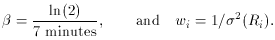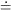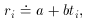### A FEW MORE ADVANCED EXAMPLES

For my first trick, let's assume that you have a radioactive sample in a physics laboratory, and with your trusty Geiger counter you want to determine the amount of some short-lived radioactive isotope present in the sample. Observing over a total span of 30 minutes, you detect the numbers of radioactive events which I have listed in Table 1-1. How much radioactive isotope was present at time t = 0, when the sample first came out of the accelerator where it had been bombarded with high-energy particles? The first thing that I want you to notice is that the raw data are simple number counts, and it is reasonable to expect that the uncertainty in any of these numbers is determined by Poisson statistics. (In fact, when I made up these data I added random Poisson noise.) Thus, we should have an excellent handle on what the observational errors ought to be. Since the raw number counts were integrated over different lengths of time, I have converted them to counting rates Ri by dividing the observed number counts Ni byt; the standard errors of these counting rates are also obtained by dividing the standard errors of the number counts byt. I suggest that we use the midpoint of each observation as the independent variable. This is not really the right thing to do (see if you can figure out why), but it is close enough for government work. Suppose we believe that our radioactive sample should consist of a pure isotope with a known half-life of seven minutes. As you know, apart from the random Poisson noise, radioactive decay follows an exponential function of time:where the parameter A simply represents the rate of decays at time t = 0 (which, of course, is proportional to the isotope's abundance, which is what we want to know), andis the natural logarithm of 2 divided by the half-life. How can we use least squares to determine the value of A, given the assumption that the half-life is seven minutes? Well, it's perfectly easy. All you have to do is recognize is that if you make the substitution x = e-t, sothen this is in exactly the same form as a problem we've seen before, that of fitting the slope of a straight line to data while forcing that line to pass through the origin. (In this case, the origin x = 0 occurs at time t =- we are requiring our solution to give zero abundance for the radioactive isotope after an infinite amount of time has passed. This is eminently reasonable.) We all remember the formula for that problem, and here it is: the best fit for the number of decays per unit time at t = 0 iswhere I have usedtime NN R(min) =N /t =N /t 0.0-1.0 1032 32.1 1032 32.1 1.0-2.0 917 30.3 917 30.3 2.0-4.0 1643 40.5 822 20.3 4.0-6.0 1416 37.6 708 18.8 6.0-8.0 1243 35.3 622 17.6 8.0-12.0 1865 43.2 466 10.8 12.0-16.0 1513 38.9 378 9.7 16.0-20.0 1210 34.8 302 8.7 20.0-24.0 967 31.1 242 7.8 24.0-30.0 1074 32.8 179 5.5

I have illustrated this fit in Fig. 1-2, both as a function of the linearized coordinate x (upper), and as a function of the actual time t = -ln(x) /(lower). As you can see, the "best" fit is a very poor one, a conclusion which is reinforced by the mean error of unit weight: if you compute the weighted mean square residual (remember, I have used s = 1), you find m.e.1 = 8.79. (Try it!) In other words, the residuals are almost nine times larger, on average, than they should be. A chi-squared test will tell you that you should never see scatter this large with standard errors like these (2 = 695 for 9 degrees of freedom). These are simple Poisson errors! We can't have got them that far wrong! The problem must be with our assumptions: our prediction that the radioactive sample should have a half-life of seven minutes was wrong.Figure 1-2

Well, then, suppose it's a pure isotope with some other, unknown, half-life? The equation describing this situation is, of course, RiAe-ti, which now has two unknown quantities, A and. Now you can't solve this equation by least squares, at least not with the techniques that I've just shown you, because the equation is not linear in the unknown parameters, A and. However, you can still make linear least squares work here by yet another change of variables. If we take the logarithm of both sides of this equation, and make three simple substitutions,then we getan equation that is linear in the two new unknowns, a and b. The only tricky thing that we have to watch out for is that the standard error of each data point must also be properly transformed to the new variables:(More on this propagation of error stuff next time. Watch for it. For now, be aware that this conversion is not mathematically perfect, in the sense that if Ri is distributed as a Gaussian random variable, ri will not be. In fact, even Ri is not distributed as a Gaussian variable, but rather as a Poisson variable, so we shouldn't really be doing least squares at all. However, as long as(R) is small compared to R, both these subtleties can be swept under the rug - they will introduce systematic errors many orders of magnitude smaller than those inherent to our small data set. Just so you know.) Now we can solve for the best estimates of a and b using the standard linear least squares that we have seen before. When we do this we getandFig. 1-3 contains two plots illustrating this fit, which obviously looks much better than the previous one, but is still not perfect. The mean error of unit weight is 2.01, or2 = 32.3 for 8 degrees of freedom. Your handy table of reduced2 values tells you that this is still very unlikely to be the correct answer: the probability of getting this value of2 if all the assumptions are correct is only about one in 104.Figure 1-3

I won't keep you in suspense any longer. I made up these data assuming that the sample was actually a mixture of three different isotopes with half-lives of 4, 7, and 23 minutes. Once you know this, you can now use linear least squares to calculate how much each of these isotopes contributes to the overall radioactivity. Once again, you have to make a certain substitution of variables to make it perfectly clear that this is in fact a problem in linear least squares, but that is not a difficulty:Let xj, i = exp(-j ti) and you haveDoes this look the least little bit familiar? Once you have turned the crank on the machinery that I set up before, you getIn other words, once you get the model right (I told you that there were three components, and I even gave you the half-lives) the mean error of unit weight comes out to a value near one, just as it should. You might like to compare these results for the number of decays due to each component to the values which I put in, to generate these data: I used A1 = 214.0, A2 = 569.0, and A3 = 321.0. As you can see, the agreement between the input and output values for the three unknowns do not show perfect agreement - after all, I did generate reasonable, random, Poisson errors for the "observations." Still, the agreement is consistent with the error bars. How did I get these error bars? I'll tell you next time.

There is one other thing that is interesting to note. The error bars on1 and2 are much larger than the error bars on3. There's a very simple explanation for this: the half-lives of 4 and 7 minutes are just too similar, given the spacing of the data points. If you look at the two rapidly decaying curves in Fig. 1-4, you can see that their shapes are very similar, and the coefficient of each is most strongly constrained by the innermost three or four data points. The shape of the slow-decaying curve is quite different, and the last three or four data points are more strongly determined by it than by the other two curves put together. In other words, the available observations do not strongly distinguish between the 4- and 7-minute half-lives: you could decrease the inferred fraction of one component, increase the fraction of the other, and not make a big difference in the quality of the fit. That is why the uncertainties in the proportions of the first two components are large. On the other hand, the abundance of the last component is well determined by the last few data points; that is why its uncertainty is small. One often hears the complaint that least-squares problems are ill-determined. That is not true of a well-designed experiment, in general. This is one of those rare, unfortunate cases, where the experiment is ill-designed, at least from the point of view of distinguishing between the first two radioactive components. Too bad the experimenter didn't start taking data 10 or 15 minutes earlier (while the sample was still in the particle accelerator?). Then the difference between the 4- and 7-minute half-lives would have been obvious.Figure 1-4

One very last point. Suppose I told you that there were three components, but I didn't give you the half-lives: could you still solve for six unknowns, three abundances and three half-lives? Ah! Now there's a problem that can't be done with linear least squares. More about that next time.